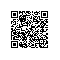# 用于 Python 的 MATLAB 引擎 API 快速入门

## 安装用于 Python 的 MATLAB 引擎 API

Matlab的官方文档中介绍了 Matlab 与其余编程语言之间的引擎接口，其中包括对于 Python 开放的引擎 API，可参考官方教程，其中包括引擎安装，基本使用，以及Python与Matlab之间的数据类型转换及交互。

• 在 Windows 系统中：(可能需要管理员权限运行)

cd "matlabroot\extern\engines\python"
python setup.py install
• 在 Mac 或 Linux 系统中：

cd "matlabroot/extern/engines/python"
python setup.py install

## 基础用法

import matlab
int_8 = matlab.int8([1, 2, 3, 4, 5, 6])
print(int_8)    # [[1, 2, 3, 4, 5, 6]]
print(int_8.size)   # (1, 6)
int_8.reshape((2, 3))   # reshape function is different from numpy
print(int_8)    # [[1, 3, 5], [2, 4, 6]]

double = matlab.double([[1, 2, 3], [4, 5, 6]])
print(double)   # [[1.0, 2.0, 3.0], [4.0, 5.0, 6.0]]
print(double)    # [1.0, 2.0, 3.0]
print(double) # 6.0

# Slice array
py = [[1, 2, 3], [4, 5, 6]]
mt = matlab.int32([[1, 2, 3], [4, 5, 6]])
py = py[::-1]
mt = mt[::-1]
# Slicing a Matlab array returns a view instead of a shallow copy
print(py)   # [[3, 2, 1], [4, 5, 6]]
print(mt)   # [[3, 2, 3], [4, 5, 6]]

Python的扩展接口 中介绍：
Python 还可以通过引擎完成对 Matlab 的一些基本操作与控制。以下代码需要在终端运行：

import matlab.engine

eng = matlab.engine.start_matlab()

print(eng.sqrt(4.))     # 2.0
eng.plot(matlab.int32([1, 2, 3, 4]), matlab.int32([1, 2, 3, 4]))

eng.eval("hold on", nargout=0)
eng.eval("plot([4, 3, 2, 1], [1, 2, 3, 4])", nargout=0)

eng.eval("x = 3", nargout=0)
eng.eval("y = 41", nargout=0)
eng.eval("z = [213, 123]", nargout=0)
print(eng.workspace)
print(eng.workspace['x'], eng.workspace['z'])
"""
Name      Size            Bytes  Class     Attributes

x         1x1                 8  double
y         1x1                 8  double
z         1x2                16  double

3.0 [[213.0,123.0]]
"""

input("Press Enter to exit.")
eng.quit()

## Python-Matlab调用（call） m 文件

• callentry.m 代码：

function [x, y, z] = callentry(a, b);
y = mul(a, b)
z = callsub(a, b)
end

function l = mul(m, n);
l=m*n;
end

l=m+n;
end
• callsub.m 代码

function r = callsub(a, b);
r = a-b;
end

import matlab.engine
eng = matlab.engine.start_matlab()
print(eng.callentry(7.7, 2.1, nargout=3))
eng.quit()

Note: 值得注意的是，此处需要设置 nargout 参数，当未设置时默认为 1，即默认只返回 1 个参数，当知道 Matlab 返回参数的数量时，通过nargout 进行设置来获取所有需要的参数。无参数返回时请设为 0

x =
9.8000

y =
16.1700

z =
5.6000

r =
9.8000   16.1700    5.6000

(9.8, 16.17, 5.6)

# MATLAB 中 调用 Python

matlab 官方教程：从 MATLAB 调用 Python

matlab 把所有参数输出到一个文件里，然后用 system 命令调 python 脚本。python 脚本读文件做计算结果再写文件。最后 matlab 再读文件得到结果。假设 python 脚本的用法是：

python xxx.py in.txt out.txt  

[status, cmdout] = system('python xxx.py in.txt out.txt')

Matlab 的 system 函数用来向操作系统发送一条指令，并得到控制台的输出，可以直接将控制台的输出在 Command Window 打印出来，或者保存在变量中。 与 system 类似的还有 dos 函数和 unix 函数，我觉得它们都是对 system 函数的一种包装，而 Matlab 的 system 函数也许是对 C 的库函数system 的包装。

## 先编写一个调用 Python 脚本的 matlab 程序即 python.m


function [result status] = python(varargin)
% call python
%命令字符串
cmdString='python';
for i = 1:nargin
thisArg = varargin{i};
if isempty(thisArg) | ~ischar(thisArg)
error(['All input arguments must be valid strings.']);
elseif exist(thisArg)==2
%这是一个在Matlab路径中的可用的文件
if isempty(dir(thisArg))
%得到完整路径
thisArg = which(thisArg);
end
elseif i==1
% 第一个参数是Python文件 - 必须是一个可用的文件
error(['Unable to find Python file: ', thisArg]);
end
% 如果thisArg中有空格，就用双引号把它括起来
if any(thisArg == ' ')
thisArg = ['"''"', thisArg, '"'];
end
% 将thisArg加在cmdString后面
cmdString = [cmdString, ' ', thisArg]
end
%发送命令
[status,result]=system(cmdString);
end  

import sys
try:
f=open(fname,'r')
cell='{'+repr(li)[1:-1]+'}'
f.close()
print cell
except IOError:
print "Can't open file "+fname
if '__main__'==__name__:
if len(sys.argv)<2:
print 'No file specified.'
sys.exit()
else:
readLines(sys.argv) 

This is test.txt
and matlab_readlines.py

### 测试：

str = python('matlab_readlines.py','test.txt');
eval(['c = ' str])
celldisp(c)使用钉钉扫一扫加入圈子
+ 订阅